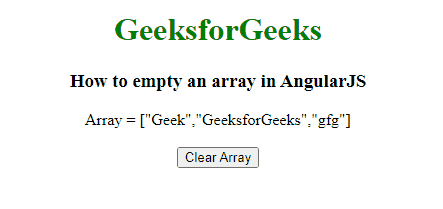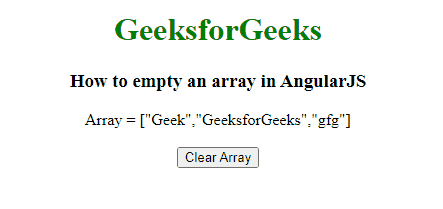# How to make empty an array using AngularJS ?

• Last Updated : 01 Aug, 2022

Given an array & the task is to make empty an array or delete all the elements from the array in AngularJS. In order to do this, there are 2 ways i.e., either use the [] notation to reinitialize the array which eventually removes all the elements from the array, or set the length of the array to 0 by using length property, which also empty the array.

We will explore both the approaches & understand them through the illustrations.

Approach: In this case. arr =[] will create a new array & assign a reference to it to a variable, while any other reference will remain unaffected that still points to the original array.

Example 1: This example illustrates empty an array using the [] notation in AngularJS.

## HTML

 ```<``html``>` `<``head``>``    ``<``script` `src``=``"//ajax.googleapis.com/ajax/libs/angularjs/1.2.13/angular.min.js"``>``    ``` `    ``<``script``>``        ``var myApp = angular.module("app", []);``        ``myApp.controller("controller", function (\$scope) {``            ``\$scope.arr = ['Geek', 'GeeksforGeeks', 'gfg'];``            ``\$scope.emptyArr = function () {``                ``\$scope.arr = [];``            ``};``        ``});``    ````` `<``body` `style``=``"text-align:center;"``>``    ``<``h1` `style``=``"color:green;"``>``        ``GeeksforGeeks``    ````    ``<``h3``>``        ``How to empty an array in AngularJS``    ````    ``<``div` `ng-app``=``"app"``>``        ``<``div` `ng-controller``=``"controller"``>``            ``Array = {{arr}}<``br``><``br``>``            ``<``button` `ng-click``=``'emptyArr()'``>``                ``Clear Array``            ````        ````    ```````

Output:Approach: In this case, arr.length = 0 manipulates the array itself, i.e., basically deleting everything from the array & while accessing the array with the help of different variables, then we will get the modified array.

Example 2: This example illustrates setting the array length to 0 using length property, which specifies an empty array.

## HTML

 ```<``html``>` `<``head``>``    ``<``script` `src``=``"//ajax.googleapis.com/ajax/libs/angularjs/1.2.13/angular.min.js"``>``    ``` `    ``<``script``>``        ``var myApp = angular.module("app", []);``        ``myApp.controller("controller", function (\$scope) {``            ``\$scope.arr = ['Geek', 'GeeksforGeeks', 'gfg'];``            ``\$scope.emptyArr = function () {``                ``\$scope.arr.length = 0;` `            ``};``        ``});``    ````` `<``body` `style``=``"text-align:center;"``>``    ``<``h1` `style``=``"color:green;"``>``        ``GeeksforGeeks``    ````    ``<``h3``>``        ``How to empty an array in AngularJS``    ````    ``<``div` `ng-app``=``"app"``>``        ``<``div` `ng-controller``=``"controller"``>``            ``Array = {{arr}}<``br``><``br``>``            ``<``button` `ng-click``=``'emptyArr()'``>``                ``Clear Array``            ````        ````    ```````

Output:My Personal Notes arrow_drop_up